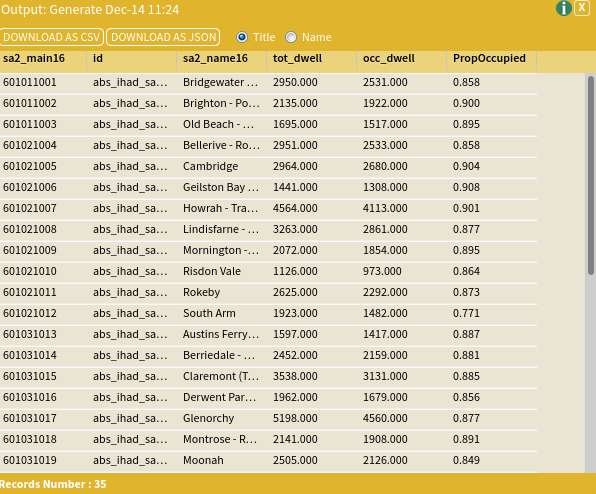Generate

The Generate tool allows you to manipulate an aggregated dataset and create new columns and variables that are the result of combining two other columns by a simple mathematical function – allowing you to calculate proportions, sums, products or differences.

Set Up

For this worked example we will calculate the proportion of total dwellings that are separate houses for SA2s across Melbourne in 2016.

To do this:

• Select Greater Hobart (gccsa_2016/6HOB) as your area
• SA2 9-Digit Code 2016
• SA2 Name 2016
• Occupied Private Dwellings (b)
• Dwellings (e)

Once you have added this dataset, you are ready to use the Generate tool – follow the inputs instructions below to see how to do this.

Inputs

We are now ready to generate a new column. In this instance, we will be working out the proportion of dwellings that occupied in Greater Hobart

To do this click the Tools button in the Analyse panel (Tools  Data Processing → Generate)Enter your parameters as shown in the image below and click the Run Tool button. These parameters are also explained below:• Dataset Input: This is the dataset that contains the columns you would like to include in the calculation. Select ABS – Index of Household Advantage and Disadvantage (IHAD) (SA2) 2016.
• Key Column: The variable indicating the key column of the dataset. Select SA2 9-Digit Code 2016.
• Operand 1: This represents the ‘left-hand side’ of the equation. Select Occupied Private Dwellings (b) column.
• Operator: This represents the mathematical function that you would like to use to create the new column. In this instance, as we are creating a proportion, select/” divide.
• Other operators include:
• – subtract
• * multiply
• / divide
• == is equal to
• != is not equal to
• < is less than > is greater than
• <= is less than or equal to
• => is greater than or equal to
• Operand 2: This represents the ‘right-hand side’ of the equation. Select Dwellings (e).
• New Column Name: This will be the new column in the output table. It is important that you only include letters, numbers and underscores (no spaces or other characters!) in this column. Also, it can only start with a letter – no number at the start! Enter PropOccupied.

Outputs

Once the tool has run, a pop-up box will appear asking you to display your results (shown below). Click Display to open the output table. You will see that there has been an entirely new table created (also shown below), which now has an additional column at the end (PropOccupied) which represents the mathematical outcome of dividing one of your original columns by another.You should now rename this dataset to something meaningful and easy to recognise, for example Houses_Proportion_Output. You can now map this output dataset and column as you normally would using the Choropleth visualisation function.

Looking for Spatial Data?

You can browse the AURIN Data Discovery:How can you Create Impact?